Skip to main content

# Counteracting ring formation in rotary kilns

## Abstract

Avoiding the formation of rings in rotary kilns is an issue of primary concern to the cement production industry. We developed a numerical combustion model that revealed that in our case study rings are typically formed in zones of maximal radiative heat transfer. This local overheating causes an overproduction of the liquid phase of the granular material, which tends to stick to the oven’s wall and to form rings. To counteract for this phenomenon, we propose to increase the amount of secondary air injected to cool the oven. Experimental validation at the plant has confirmed that our solution is indeed effective. For the first time in years, the kiln has been operating without unscheduled shut-downs, resulting in hugely important cost savings.

## 1 Introduction

### 1.1 Rotary kilns

Rotary kilns  are long cylindrical industrial furnaces that for their operation are slightly tilted as shown in Figure 1. They are used in a wide range of material processing industries. The material to be processed is fed into the upper end of the cylinder, leaving a considerable amount of freeboard or empty space. As the kiln rotates about its axis, the material bed gradually moves down towards the lower end, and undergoes a certain amount of stirring and mixing. The material leaves the oven at the lower end to be further processed. The heating of the kiln serves to drive the specific bed reactions, which, for either kinetic and thermodynamic reasons, require high temperatures. The CFD simulation of these devices has been considered in e.g. .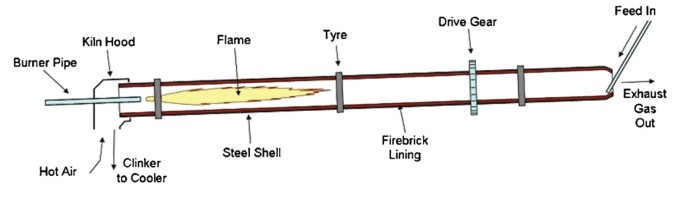Figure 1

We will look into direct fired kilns in which the energy necessary to heat the material to the level required for the intended reactions is generated by the combustion of hydrocarbon fuels continuously fed to a burner placed in the freeboard. This energy is subsequently transferred by heat exchange between the gas phase and the material bed. The lateral surface of the kiln is covered by an isolating lining of refractory material. Hot gases flow in a direction opposite to that of the material bed through the kiln. The heat transfer between the freeboard and the bed is a rather complex phenomenon as it occurs along various paths determined by the physics of radiation. Various transport processes therefore constitute the working principle of the ovens considered.

We study a rotary kiln used by Almatis B.V. in Rotterdam for a production of calcium-aluminate cement, a white, high purity hydraulic bonding agent providing controlled setting times and strength required in today’s high performance refractories operating at temperatures up to $1,800$ degrees Celsius. This cement is made by fusing a mixture of a calcium-bearing limestone and an aluminum-bearing material. The following two considerations will be important in our approach to the modeling of the kiln. The first is that the material bed occupies no more than five percent of the total volume of the kiln. The second is that the combustion of fuel by the burner is aided by inflow of preheated air through a secondary air inlet.

### 1.2 Ring formation

As material slides and tumbles slowly through a heated rotary kiln, a thin layer of dust invariably forms on its inner lateral surface. Some zones of the kiln may be particularly prone to particle accumulation in such a way that the combination of particular thermal and flow conditions results in the formation of cylindrical deposits of solid crystalline structures referred to as rings. As such rings grow thicker, they form a dam in the freeboard, hindering the flow of material and flue gasses through the kiln.

In the kiln we consider, we observed in particular so-called front-end/mid-kiln rings . They are formed close to the burner and are presumably caused by the direct impingement of the burner flame on the lateral surface causing local overheating. These are the most common and most troublesome type of rings as they cannot be reached from the kiln’s exterior and are therefore impossible to remove while the kiln is in operation. In severe cases, ring dams grow rapidly and cause the unscheduled shutdown of the kiln. In the last three years we registered on average at least one ring formation per month, of which seventy percent caused the unforeseen shutdown of the kiln for on average three days. Each of these kiln outages causes very important production and turnover losses.

This paper is structured as follows. In Section 2 we motivate the development of a computational fluid dynamics model for the kiln. In Section 3 we give details of this model’s operational setup and geometry, the polyhedral mesh discretizing the spatial coordinates and the partial differential equations governing the gas flow in the oven, the combustion of hydrocarbon fuel in the burner, the reactions of the chemical species and the radiative heat transfer in the freeboard. In Section 4 we present numerical results that convincingly show that our model allows to localize and counteract the ring formation by increasing the amount of air through the secondary air inlet. In Section 5 finally we report on an experimental validation at the plant that confirms the effectiveness of the change in operating conditions that we propose.

## 2 Localizing and counteracting ring formation

Experience at the plant has shown that neither trial and error nor simplified mathematical modeling approaches provide sufficient insight to eliminate the problem of ring formation. Measuring the temperature inside the kiln, using thermocouples for instance, has proven to be difficult due to the harsh operating conditions. We therefore decided to develop a sufficiently detailed mathematical model enabling to link the ring formation to the temperature, radiative heat and gas flow profiles in the kiln. This model will be described in more details in the next section and has revealed that rings are typically formed in zones of maximal radiative heat transfer. In a phase-diagram this local overheating can be linked to the overproduction of the amount of liquid phase of the material. Such a phase diagram is shown in Figure 2 for one of the raw materials to be processed.Figure 2

The insight linking the ring formation to local overheating proved to be instrumental in altering the operating conditions of the kiln in such a way to avoid ring formation. Our solution avoids drastic changes in the geometry of the kiln, in the configuration of the burner and in the properties of the fuel or the lining. We instead alter the fuel/air composition by altering the amount of preheated air injected through the secondary air inlet shown in Figure 3. This allows us to eliminate peaks in temperature and radiative heat transfer profiles, to limit the amount of liquid phase production and therefore avoid ring formation. The change in operating conditions we propose does maintain the temperature distribution required by the stringent quality specifications on the end product.Figure 3

## 3 Computational fluid dynamics model

The detailed mathematical model we developed is a multi-physics model that takes the following phenomena into account: the reactive gas flow and temperature, chemical species and radiative heat transfer distribution in the kiln, the turbulent non-premixed combustion of hydro-carbon gasses in the burner, the insulating properties of the lining, the rotary motion of the kiln and the forced convection on the outside surface.

The material bed only occupies a small fraction of the volume of the kiln and has a negligible limited impact of temperature distribution. We therefore do not take the material bed into account in our model and simulate an empty kiln.

In the kiln hot gases are generated by a flame projected from a burner-pipe placed inside the freeboard. The setup of the burner is shown in Figure 3 and Figure 4. The burner injects fuel in axial and radial direction and is cooled by an amount of air forced through the cooling slots. The flow of cooling air through the rectangular air inlet breaks the circular symmetry of the configuration and requires a model in three spatial dimensions to be resolved.Figure 4

The most important physical phenomenon that takes place in this burner region is the turbulent non-premixed combustion of the fuel injected from the burner with the secondary air. Combustion, even without turbulence, is an intrinsically complex process involving a large range of chemical time and length scales. Some of the chemical phenomena controlling flames take place in short times over thin layers and are associated with very large mass fractions, temperature and density gradients. The full description of chemical mechanisms in laminar flames may require hundreds of species and thousands of reactions leading to considerable numerical difficulties. Turbulence itself is probably the most complex phenomenon in non-reacting fluid mechanics. Various time and length scales are involved and the description of turbulence remains to date an open questions. The modeling of the kiln therefore requires resorting to a set of assumptions that are described in the remainder of this section.

### 3.1 The geometry

The geometry model of the kiln is shown in Figure 3. Figure 3(a) and Figure 3(b) give an exterior view on the complete kiln and a more detailed interior view of the burner region, respectively. The challenging aspect of this geometry is that the inlets of the burner are a factor thousand times smaller than the axial length of the kiln, imposing challenges in the mesh generation process.

### 3.2 Grid generation

The mesh model is shown in Figure 4. Figure 4(a), Figure 4(b) and Figure 4(c) give an exterior view of the mesh in the air inlet region, an interior view of the mesh and a detailed view of the mesh on the burner, respectively. We employed a polyhedral mesh of 2.8 million cells with local refinement in the critical inlets and burner regions. The main difficulties in meshing this geometry were found in balancing the accuracy required capture the flow around small features in the burner with the overall computational cost. Polyhedral meshes provide a balanced solution in complex mesh generation problems of this kind.

Tetrahedra are the simplest type of volume elements. As their faces are plane segments, both face and volume centroid locations are well defined. A disadvantage is that tetrahedra cannot be stretched too much. To achieve a reasonable accuracy a much larger number of control volumes is needed than if structured meshes are used. Furthermore, as tetrahedral control volumes have only four neighbors, and computing gradients at cell centers using standard approximations can be problematic.

Polyhedra offer the same automatic meshing benefits as tetrahedra while overcoming their disadvantages. A major advantage of polyhedral cells is that they have many neighbors (typically of order 10) allowing gradients to be much better approximated. Obviously more neighbors implies more storage and computational operations per cell, but this is more than compensated by a higher accuracy. Polyhedral cells are also less sensitive to stretching than tetrahedra. A polyhedron with 12 faces for instance has six optimal directions which, together with the larger number of neighbors, leads to a more accurate solution with a lower cell count. Comparisons in many practical tests have verified that with polyhedral meshes, one needs about four times fewer cells, half the amount of memory and a tenth to a fifth of computing time compared to tetrahedral meshes to reach solutions of the same accuracy. In addition, solvers on polyhedral meshes were found to converge more robustly with respect to change in their parameters. A more detailed analysis of polyhedral meshes can be found in .

### 3.3 Governing reacting flow equations

In this section we present the conservation equations for reacting flows we used. The equations are derived from the Navier-Stokes (NS) equations by adding terms that account for reacting flows. The reacting gas is a non-isothermal mixture of multiple species which must be tracked individually. As heat capacities change significantly with temperature and composition, the transport coefficients require specific attention. In this subsection we will describe the Navier-Stokes and Reynolds-Averaged Navier-Stokes flow model, the non-realizable K-Epsilon turbulence model and the Standard Eddy Break Up combustion model. A more detailed derivation of these equations can be found in e.g. [7, 8].

Species are defined by their mass fraction defined as

(1)

where N is the number of species in the reacting mixture, $m ℓ$ the mass of species in a volume V and m the mass of gas in the volume, respectively. The conservation of mass can then be written as

NS: Conservation of mass

$D ρ D t = ∂ ρ ∂ t + ∂ ρ u i ∂ x i =0,$
(2)

where $ρ=m/V$ is the density of the gas and $u i$ its three dimensional velocity field, respectively. The conservation of species for $ℓ=1:N$ can then be written as

NS: Conservation of species

$∂ ρ Y ℓ ∂ t + ∂ ∂ x i ( ρ ( u i + V ℓ , i ) Y ℓ ) = ω ˙ ℓ ,$
(3)

where $V ℓ , i$ the i th component of the diffusion velocity $V ℓ$ of species and $ω ˙ ℓ$ the chemical reaction rate of species . The conservation of momentum for the gas can for $j=1:3$ be expressed as:

NS: Conservation of momentum

$∂ ∂ t ( ρ u j ) + ∂ ∂ x i ( ρ u i u j ) = ∂ σ i j ∂ x i + ρ ∑ ℓ = 1 N Y ℓ f ℓ , j = − ∂ p ∂ x j + ∂ τ i j ∂ x i + ρ ∑ ℓ = 1 N Y ℓ f ℓ , j ,$
(4)

where p denotes pressure and $τ i j$ and $f ℓ , j$ are the components of the Reynolds stress tensor and of the volume force acting on species , respectively. We will express the conservation of energy using the sensible enthalpy of the mixture $h s$ as independent variable. To introduce this quantify, we will first denote the enthalpy of species as $h ℓ$. This quantify is the sum of the of a sensible and chemical part, i.e.,

$h ℓ = h ℓ , s +Δ h f , ℓ 0 ,$
(5)

where the last term represents the enthalpy of formation of the species at a particular reference temperature $T 0$. The enthalpy of the mixture is then defined as a weighted average that again can be decomposed into a sensible and chemical part, as follows

$h = ∑ ℓ = 1 N Y ℓ h ℓ = ∑ ℓ = 1 N Y ℓ h ℓ , s + ∑ ℓ = 1 N Y ℓ Δ h f , ℓ 0 = h s + ∑ ℓ = 1 N Y ℓ Δ h f , ℓ 0 .$
(6)

We will denote the heat diffusion coefficient and the temperature by λ and T, respectively. The energy flux $q i$ is the sum a heat diffusion term derived from Fourier’s Law and a term associated with the diffusion of species with different enthalpies, i.e.,

$q i =−λ ∂ T ∂ x i +ρ ∑ ℓ = 1 N h ℓ Y ℓ V ℓ , i .$
(7)

In the energy conservation equation the following three source terms will play a role: the heat source due to radiative heat flux denoted as Q, the viscous heating term denoted as Φ, where $Φ= τ i j ∂ u i ∂ x j$ and heat release due to combustion denoted as $ω ˙ T$, where

$ω ˙ T =− ∑ ℓ = 1 N Δ h f , ℓ 0 ω ˙ ℓ .$
(8)

The work done by the gas on the species can be expressed as $ρ ∑ ℓ = 1 N Y ℓ f ℓ , i V ℓ , i$. With all these quantities introduced, the conservation of energy in terms of $h s$ can be expressed as:

NS: Conservation of energy

$ρ D h s D t = ω ˙ T + D p D t − ∂ ∂ x i ( λ ∂ T ∂ x i ) + Φ + Q ˙ − ∂ ∂ x i ( ρ ∑ ℓ = 1 N h s , ℓ Y ℓ V ℓ , i ) + ρ ∑ ℓ = 1 N Y ℓ f ℓ , i V ℓ , i .$
(9)

Turbulent combustion results from the two-way interaction between chemistry and turbulence. When a flame interacts with a turbulent flow, the combustion modifies the turbulence in two ways. The heat released induces high flow accelerations through the flame front and the temperature changes generate large changes in kinematic viscosity. These phenomena may either generate or damp turbulence and are referred to as flame-generated turbulence and relaminarization due to combustion, respectively. The turbulence conversely modifies the flame structure. This may either enhance the chemical reactions or completely inhibit it, leading to flame quenching. Compared to premixed flames, turbulent non-premixed flames exhibit some specific features that have to be taken into account. Non-premixed flames do not propagate as they localized on the fuel-oxidizer interface. This property is useful for safety purposes but it also has consequences on the chemistry-turbulence interaction. Without propagation speed, a non-premixed flame is unable to impose its own dynamics on the flow field and is therefore more sensitive to turbulence.

The description of the turbulent non-premixed combustion processes in a computational fluid dynamics model may be achieved using three levels of accuracy in the computations. Either a Reynolds Averaged Navier Stokes (RANS), a Large Eddy Simulations (LES) or a Direct Numerical Simulations (DNS) model can be adopted. In current engineering practice, the RANS model is extensively used because it is less demanding in terms of resources. Its validity however is limited by the closure models describing turbulence and combustion and the need for some form of callibration. Considering the complexities and the dimensions of our kiln, using the RANS model is the only feasible choice.

#### 3.3.1 RANS model

In constant density flows, Reynolds averaging consist in splitting any quantity ξ in mean and fluctuating component ($ξ= ξ ¯ + ξ ′$). In variable density flow Favre  mass-weighted averages are usually preferred, i.e., $f ˜ = ρ f ¯ ρ ¯$. Any quantity f can therefore be split into:

The RANS equations derived from the reacting Navier-Stokes equation given above are then given by the equation for conservation of mass

RANS: Conservation of mass

$∂ ρ ¯ ∂ t + ∂ ρ ¯ u i ˜ ∂ x i =0,$
(10)

the equation for conservation of species for $ℓ=1:N$

RANS: Conservation of species

$∂ ∂ t ( ρ ¯ Y ℓ ˜ )+ ∂ ∂ x i ( ρ ¯ u i ˜ Y ℓ ˜ )= ω ˙ ¯ ℓ − ∂ ∂ x i ( V ℓ , i Y ℓ ¯ + ρ ¯ u i ″ Y ℓ ″ ˜ ) ,$
(11)

the equation for conservation of momentum for $j=1:3$

RANS: Conservation of momentum

$∂ ∂ t ( ρ ¯ u j ˜ )+ ∂ ∂ x i ( ρ ¯ u i ˜ u j ˜ )+ ∂ p ¯ ∂ x j = ∂ ∂ x i ( τ i j ¯ − ρ ¯ u i ″ u j ″ ˜ ) ,$
(12)

and finally the equation for conservation of momentum

RANS: Conservation of energy

$∂ ∂ t ( ρ ¯ h s ˜ ) + ∂ ∂ x i ( ρ ¯ u i ˜ h s ˜ ) = ω ˙ ¯ T + D p ¯ D t + ∂ ∂ x i ( λ ∂ T ∂ x i ¯ − ρ ¯ u i ″ h s ″ ˜ ) + Φ ¯ − ∂ ∂ x i ( ρ ∑ k = 1 N h s , ℓ Y ℓ V ℓ , i ¯ ) .$
(13)

The averaging procedure introduces unclosed quantities that have to be modeled. Without entering in the details we list here the two main unclosed terms that will be described in the next sections:

• Reynolds stresses: $ρ ¯ u i ″ u j ″ ˜$.

• Species chemical reaction rates: $ω ˙ ¯ ℓ$.

#### 3.3.2 Turbulence model

Using the turbulence viscosity assumption by Boussinesq , the Reynolds stresses can be represented as

$ρ u i ″ u j ″ ¯ = ρ ¯ u i ″ u j ″ ˜ = − μ t ( ∂ u ˜ i ∂ x j + ∂ u ˜ j ∂ x i − 2 3 δ i j ∂ u ˜ K ∂ x K ) + 2 3 ρ ¯ k ,$
(14)

where $μ t = ρ ¯ ν t$ is the turbulent dynamic viscosity and $δ i j$ the Kronecker delta. The turbulent kinetic energy k in turn can be expressed as

$k= 1 2 ∑ j = 1 3 u j ″ u j ″ ˜ .$
(15)

Modeling the turbulent viscosity $μ t$ is the central problem in turbulence computations. Many approaches exist. In this work we use a classical turbulence model developed for non-reacting flows, namely the Realizable K-Epsilon model . Heat release effects on the Reynolds stresses are not explicitly taken into account in this approach and the turbulent viscosity is modeled as

$μ t = ρ ¯ C μ k 2 ε ,$
(16)

where ε is the rate of energy dissipation. In this model the critical coefficient $C μ$ is a function of mean flow and turbulence properties, rather than assumed to be constant as in the standard model. This allows to satisfy certain mathematical constraints on the normal stresses consistent with the physics of turbulence and is referred to as realizability.

From the Boussinesq relationship in Equation (14) and the eddy viscosity definition in Equation (16) it is possible to obtain the following expression for the normal Reynolds stress $u 2 ¯$ in an incompressible strained mean flow U

$u 2 ¯ = 2 3 k−2 ν t ∂ U ∂ x ,$
(17)

where $ν t = μ t ρ$. It can be shown that $u 2 ¯$, which by definition is a positive quantity, become negative (non-realizable), when the strain is large enough to satisfy

$k ε ∂ U ∂ x > 1 3 C μ ≈3.7.$
(18)

The easiest way to ensure the realizability is to make $C μ$ in (16) variable .

The critical coefficient $C μ$ can be expressed as a function of the mean strain and rotation rates, the angular velocity of the system rotation and the turbulence fields as follows

$C μ = 1 A 0 + A s k U ∗ ε ,$
(19)

where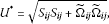(20)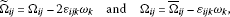(21)

where $Ω ¯ i j$ is the mean rate of rotation tensor viewed in a rotating reference frame with the angular velocity $ω k$. The parameters $A 0$ and $A s$ in (19) can be computed as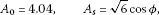(22)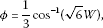(23)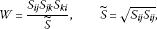(24)(25)

The turbulent kinetic energy k and its dissipation rate ε in Equation (16) are described by the following two balance equations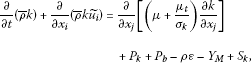(26)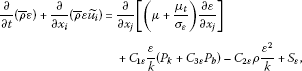(27)

where $P k$ is the production term of turbulent kinetic energy due to the mean velocity gradients, $P b$ the production of turbulent kinetic energy due to buoyancy, $Y M$ the dilatation dissipation term that accounts for the contribution of the fluctuating dilatation in compressible turbulence to the overall dissipation rate, $S k$ and $S ε$ user defined source terms for turbulent kinetic energy and dissipation, and $σ k$ and $σ ε$ the turbulent Prandtl numbers for k and ε, respectively. $C 1 ε$, $C 2 ε$ and $C 3 ε$ are model constants.

Another weakness of traditional K-Epsilon turbulence models is their modeling of the dissipation rate ε. Indeed, the well-known spreading (or dispersion) rate anomaly refers to the fact that traditional models do reasonably well in predicting the spreading rate of a planar jet but perform unexpectedly poor for rounds jets. This weakness can be traced back to a deficiency in traditional ε-equations. The realizable model proposed by Shih  was developed to repair this deficiency and addresses as such an issue that is of primary importance in our study.

#### 3.3.3 Combustion model

The averaged equation for conservation of species (11) can be rewritten in compact form for $ℓ=1:N$ as

$∂ ∂ t ( ρ ¯ Y ℓ ˜ )+ ∂ ∂ x i ( ρ ¯ u i ˜ Y ℓ ˜ )=−∇⋅ J ℓ + ω ˙ ¯ ℓ ,$
(28)

where $J ℓ$ is the mass diffusion flux of species . The previous equation is solved in a CFD code for $N−1$ species where N is the total number of fluid phase chemical species present in the system. Since the mass fraction of the species must sum to unity, the N th mass fraction is determined as one minus the sum of the $N−1$ solved mass fractions. To minimize numerical error, the N th species should be selected as that species with the overall largest mass fraction.

In turbulent flows the mass diffusion flux is computed as

$J ℓ =− ( ρ ¯ D ℓ + μ t Sc t ) ,$
(29)

where $Sc t$ is the turbulent Schmidt number and $D ℓ$ is the molecular diffusivity of species .

The species chemical reaction rate unclosed term $ω ˙ ℓ$ must be modeled with a combustion model. A combustion model describes the two-way interaction between properties of the turbulent flow produced by the flame and the chemical reactions. It serves to compute the reaction state space and the quantities it influences, namely the fluid density, viscosity, and temperature. It accounts for the processes that occur at length and time scales that we cannot resolve on a grid either in space or time due to limitations in computational resources. The choice of combustion model is decided by knowing the Damkohler number, defined as $Da= t mix t rxn$, where $t mix$ is the mixing time scale and $t rxn$ is the reaction time scale. When the Damkohler number is very large, as in the case of the kiln, the reaction rate is controlled by the turbulent mixing that brings reactants together at the molecular scale. In this limit, the Standard Eddy Break Up (EBU)  model is fairly accurate because it assumes that the reaction occurs instantaneously upon micromixing.

The EBU combustion model tracks individual mean species concentrations on the grid through transport equations. The reaction rates used in these equations are calculated as functions of the mean species concentrations, turbulence characteristics and, depending on the specific model used, temperature. A mean enthalpy equation is solved in addition to the species transport equations. The mean temperature, density and viscosity are then calculated knowing the mean enthalpy and species concentrations. In the EBU used, the individual species in the global reaction are assumed to be transported at different rates according to their own governing equations.

The reaction rate is modeled through an expression that takes the turbulent micromixing process into account. This is done through dimensional arguments. Thus, for a reaction of the form

$v F F+ v O O⟶ v P 1 P 1 + v P 2 P 2 +⋯+ v P j P j ,$
(30)

where F stands for fuel, O oxidiser and P products of the reaction, the reaction rate for reaction is assumed to be

$ω ˙ ¯ ℓ = ρ ¯ M ( 1 τ mix ) A ebu min { Y ¯ F , Y ¯ O s O , B ebu ( Y ¯ P 1 s P 1 + ⋯ + Y ¯ P j s P j ) } ,$
(31)

where $s O = v O M O v F M F$, $s P j = | v P j | M P j v F M F$, v is the molar stoichiometric coefficient for species j in reaction , M is molecular weight of species. Equation (30) essentially states that the integrated micromixing rate is proportional to the mean (macroscopic) concentration of the limiting reactant divided by the time scale of the large eddies ($k ε = τ mix$). $Y ¯ F$, $Y ¯ O$, $Y ¯ P$ are respectively the mean concentrations of fuel, oxidizer, and products. $A ebu$ and $B ebu$ are model constants with typical values of 0.5 and 4.0 respectively. The values of these constants are fitted according to experimental results and they are suitable for most cases of general interest.

In our simulations we used a reduced combustion mechanism with 6 species and 4 reactions to account for a fuel that is a mixture of different alkanes. This mixture consists for 95% of CH4 and for 5% of C2H6, C3H8 and C4H10.

The above models are discretized by a finite volume technique using second order upwinding for the convective terms . The flow equations are solved in a segregated approach in which the SIMPLE algorithm realizes the velocity-pressure coupling. The energy equation is solved for the chemical thermal enthalpy using again a segregated approach. The temperature is computed from the enthalpy according to the equation of state. At each outer non-linear iteration the resulting linear systems are solved using an algebraic multigrid preconditioner for a suitable Krylov subspace acceleration .

### 3.4 Additional information

In situations in which the media separating hot walls is transparent for thermal radiation as in the case of dry air, radiation can only occur as a surface phenomenon. In the our case however, the gas in the freeboard of the kiln will absorb, emit and scatter the thermal radiation intensity emitted from the hot walls of the kiln. This process is governed by the radiative transfer equation (RTE) that is implemented in a Participating Media Radiation Model. The model is discretized in solid angle by the discrete ordinate method described in detail in [18, 19].

### 3.5 Software implementation

Simulations were performed using the STAR-CCM+ software suite  on a ten-nodes Linux cluster having Intel Duo and Quad Core processors at a clock speed between 2.20 GHz and 3.16 GHz running a Slackware 13 64-bit distribution. Iterating the three-dimensional combustion model to equilibrium state required between $3,500$ and $4,000$ non-linear iterations and between three and three and a half days of computation time.

## 4 Computational results

In this section we discuss how our combustion model validates the difference between two operating conditions of the oven. These operating conditions are listed in Table 1 and differ in the amount of preheated air that flows through the secondary air inlet shown in Figure 3. In the first and second line in Table 1 the ratio of volume air to volume of fuel equals nine and twelve, respectively. The ratio of nine corresponds to the kiln’s operation conditions that were commonly used before the start of this work and will be referred to as the standard operating conditions. The ratio of twelve correspond to the new operation conditions that we propose. In switching from the standard to the new configuration, the flux of fuel injected in axial and radial direction and the flux of air through the cooling slot is kept the same. The effect of this switch in operation conditions on the mass of oxygen and methane is shown in Figure 5. This figure shows that with in the new operating conditions the oxigen is transported further down in the kiln.Figure 5

The objective of this section is to show how according to the combustion model described in the previous section the new configuration is less prone to the development of rings than the standard configuration. The two key arguments in this demonstration are the temperature and radiative heat distribution on the inside wall of the kiln. These will be elaborated separately in the two subsections.

### 4.1 Effect on temperature

In Figure 6, Figure 7 and Figure 8 we show how the change in operating conditions affects the computed temperature profile. Figure 6 shows the temperature distribution on an axial section through the air inlet. Figure 7 shows the temperature evaluated at the centroid of each cell of the surface mesh on the inside wall versus the centroid’s axial distance. This wall forms the interface between the hot gas and the innermost part of the insulating lining. For positions closer to low end of the kiln the temperature values shown vary more with the circumferential angle due to the higher temperature gradients caused by the injected air. The lines in Figure 7 are therefore thicker for small values of the abscis. Figure 8 finally shows the temperature distribution on the inside wall of the kiln. In this figure the flame propagating from the burner is represented by an iso-surface that encloses a region in which the methane concentration is larger than or equal to 0.01. The vertical and horizontal color bars indicate the temperature of the flame and the wall, respectively.Figure 6Figure 7Figure 8

Figure 6 clearly illustrates the effect of switching to the new operating conditions as it shows in the new conditions, the relatively cold preheated air is transported further into the kiln acting as a coolant on the wall. Figure 7 shows that this coolant reduces the wall peak temperature drops by 3.5% from $1,964$ degrees Celcius to $1,894$ degrees Celcius. It also shows that switching to the new operating conditions does not significantly alter the global distribution characteristics of the temperature which is of paramount importance in the material production process. Figure 8 is particularly interesting as it shows that in both configurations the peak in temperature is situated in a zone at four and halve to seven meters from the burner. This zone coincides with the zone in which rings are typically formed. It is therefore plausible that a reduction in peak temperature by switching to the new operating conditions will result in a reduction of the amount of liquid phase of the material being formed, and therefore impede the formation of rings. This hypothesis will be confirmed by looking into the computed radiative heat transfer distribution as we will do in the next subsection.

### 4.2 Effect on radiative heat transfer

In Figure 9, Figure 10 and Figure 11 and we show how the change in operating conditions affects the computed radiative heat transfer on the inside wall of the kiln. Figure 9 and Figure 10 are the equivalent for radiative heat transfer of Figure 7 and Figure 8 for the temperature, respectively. Figure 11 finally gives an view on the radiative heat transfer distribution from the inside of the kiln.Figure 9Figure 10Figure 11

Figure 9 confirms that as expected changing to the new operating conditions results in a lowering of the peak in radiative heat transfer. Figure 10 shows that peak in the radiative heat transfer drops by 13.5% from $5,718,029$ W/m2 to $5,062,986$ W/m2 and that the region of high values is reduced in size. Figure 11 gives another representation of this fact. By switching to the new operating conditions, the material in the kiln is less likely to absorb an excessive amount of heat, effectively limiting the amount of liquid phase of the material and therefore the formation of rings.

## 5 Experimental validation at the plant

The change in operating conditions we propose was experimentally validated at the plant for the first time on August 28th, 2011. That day the formation of a massive ring was reported. After assessing the situation, it was decided to change to the new operating conditions. The effect of this decision over time is shown in Figure 12. Four hours after the decision was taken, it was observed that the ring stopped growing in size as shown in Figure 12(a). After twenty-four hours, the ring started to break into lumps due to the vibration of the drive gears as shown in Figure 12(b). These lumps are transported through the kiln and leave it at the lower end by the rotary motion. After forty hours, the kiln is clean and in stable operating conditions as shown in Figure 12(c). Careful analysis has revealed that with the new operating conditions, the final cement product still meets the stringent quality specifications. After August 28th, 2011 the benefits of the changing to the new operating conditions has been repeatedly observed when maintenance of other circumstances required switching back to the standard operating conditions.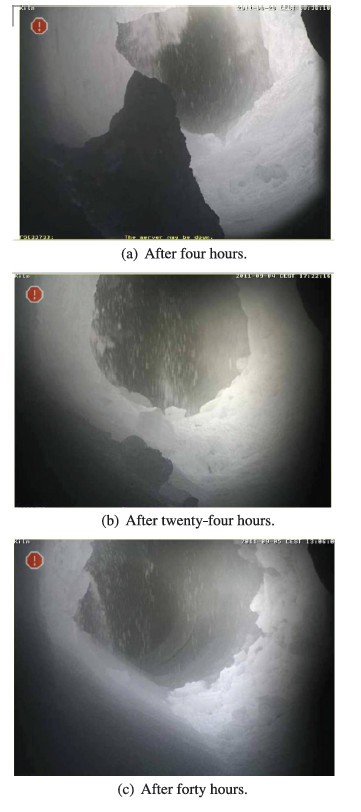Figure 12

## 6 Conclusions

We developed a numerical model allowing to access the effectiveness of measures implemented to counteract the formation of rings in a rotary cement kiln in use by Almatis B.V. in Rotterdam. In this three-dimensional combustion model, the gas flow, the temperature profile, radiative heat distribution and the concentration of hydro-carbon species in the kiln is taken into account. Simulations show that deluting the air-fuel mixture with air reduces peaks in radiative heat transfer in zones critical to ring formation. This reduction results in turn in less heat being absorbed by the granular material bed, effectively reducing the amount of material liquid phase prone to sticking to the kiln’s surface and to forming rings. The validity of our model has been experimental observed at the Almatis plant in Rotterdam. Since August 28th, 2011, the kiln has been operation without unscheduled shut-downs, resulting in hugely important cost savings.

## References

1. 1.

Boateng AA: Rotary Kilns: Transport Phenomena and Transport Processes. Butterworth-Heinemann, Stoneham; 2006.

2. 2.

Mastorakos E, Massias A, Tsakiroglou CD, Goussis DA, Burganos VN, Payatakes AC: CFD predictions for cement kilns including flame modelling, heat transfer and clinker chemistry. Appl Math Model 1999,23(1):55–76. 10.1016/S0307-904X(98)10053-7

3. 3.

Marias F, Roustan H, Pichat A: Modelling of a rotary kiln for the pyrolysis of aluminium waste. Chem Eng Sci 2005,60(16):4609–4622. 10.1016/j.ces.2005.03.025

4. 4.

Giannopoulos D, Kolaitis DI, Togkalidou A, Skevis G, Founti MA: Quantification of emissions from the co-incineration of cutting oil emulsions in cement plants: part i: NO x , CO and VOC. Fuel 2007,86(7–8):1144–1152. 10.1016/j.fuel.2006.10.025

5. 5.

Tran HN, Barham D: An overview of ring formation in lime kilns. Tappi J 1991,74(1):131–136.

6. 6.

Peric M: Flow simulation using control volumes of arbitrary polyhedral shape. ERCOFTAC Bulletin September 2004, 62. Peric M: Flow simulation using control volumes of arbitrary polyhedral shape. ERCOFTAC Bulletin September 2004, 62.

7. 7.

Williams FA: Combustion Theory. 2nd edition. Westview Press, New York; 1994.

8. 8.

Kuo KK: Principles of Combustion. 2nd edition. Wiley-Interscience, New York; 2005.

9. 9.

Poinsot T, Veynante D: Theoretical and Numerical Combustion. 2nd edition. Edwards, Ann Arbor; 2005.

10. 10.

Hinze JO: Turbulence. McGraw-Hill, New York; 1975.

11. 11.

Shih TH, Liou WW, Shabbir A, Zhu J: A new k - ϵ Eddy-viscosity model for high Reynolds number turbulent flows - model development and validation. Comput Fluids 1995,24(3):227–238. 10.1016/0045-7930(94)00032-T

12. 12.

Reynolds WC Lecture Notes for Von Karman Institute. Fundamentals of Turbulence for Turbulence Modeling and Simulation 1987. Agard Report No. 755. Agard Report No. 755.

13. 13.

Magnussen BF, Mjertager BH: On mathematical modeling of turbulent combustion. In 16th Symp. (Int.) on Combustion. The Combustion Institute, Pittsburgh; 1976:719–727.

14. 14.

Demirdzic I, Peric M: Finite volume method for prediction of fluid flow in arbitrarily shaped domains with moving boundaries. Int J Numer Methods Fluids 1990,10(7):771–790. 10.1002/fld.1650100705

15. 15.

Mathur SR, Murthy JY: A pressure-based method for ustructured meshes. Numer Heat Transf, Part B, Fundam 1997,31(2):195–215. 10.1080/10407799708915105

16. 16.

Murthy JY, Mathur SR: Radiative heat transfer in axisymmetric geometries using an unstructured finite-volume method. Numer Heat Transf, Part B, Fundam 1998,33(4):397–416. 10.1080/10407799808915040

17. 17.

Trottenberg U, Oosterlee C, Schüller A: Multigrid. Academic Press, San Diego; 2001.

18. 18.

Modest MF: Radiative Heat Transfer. McGraw-Hill, New York; 1993.

19. 19.

Siegel R, Howell JR: Thermal Radiation Heat Transfer. 3rd edition. Hemisphere, Washington; 1992.

20. 20.

Star-CCM+ Version 6.06.011 user guide; 2011. Star-CCM+Version 6.06.011 user guide; 2011.

Download references

## Acknowledgements

We thank Marco Talice for his advice worth more than platina and the CD-ADAPCO London office for the support in using their software.

## Author information

Authors

### Corresponding author

Correspondence to D Lahaye.

## Additional information

### Competing interests

The authors declare that they have no competing interests.

### Authors’ contributions

MP developed and validated the CFD model. RS provided supervision from within the industrial partner. DL provided supervision from within the academic partner.

## Authors’ original submitted files for images

Below are the links to the authors’ original submitted files for images.

## Rights and permissions

Reprints and Permissions

## About this article

### Cite this article

Pisaroni, M., Sadi, R. & Lahaye, D. Counteracting ring formation in rotary kilns. J.Math.Industry 2, 3 (2012). https://doi.org/10.1186/2190-5983-2-3

Download citation

• Received:

• Accepted:

• Published:

### Keywords

• rotary kiln
• computational fluid dynamics
• ring formation